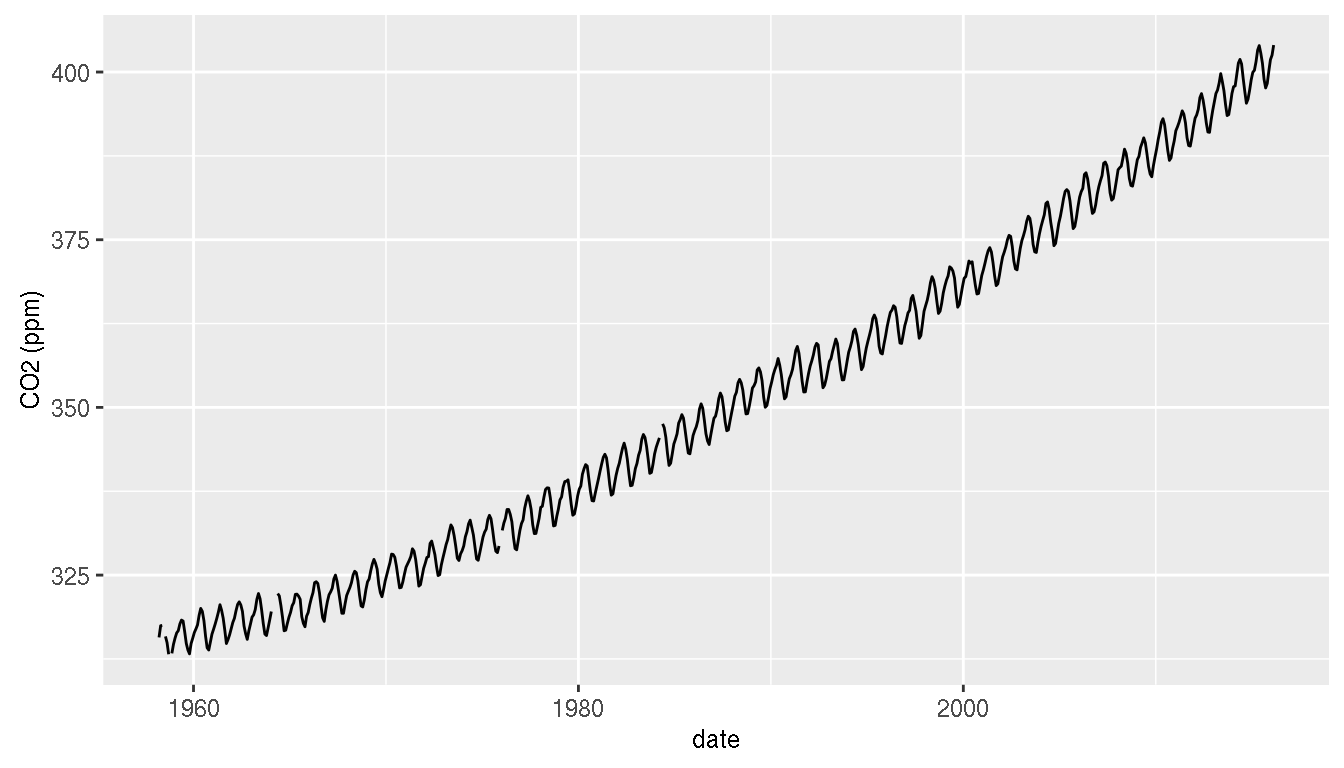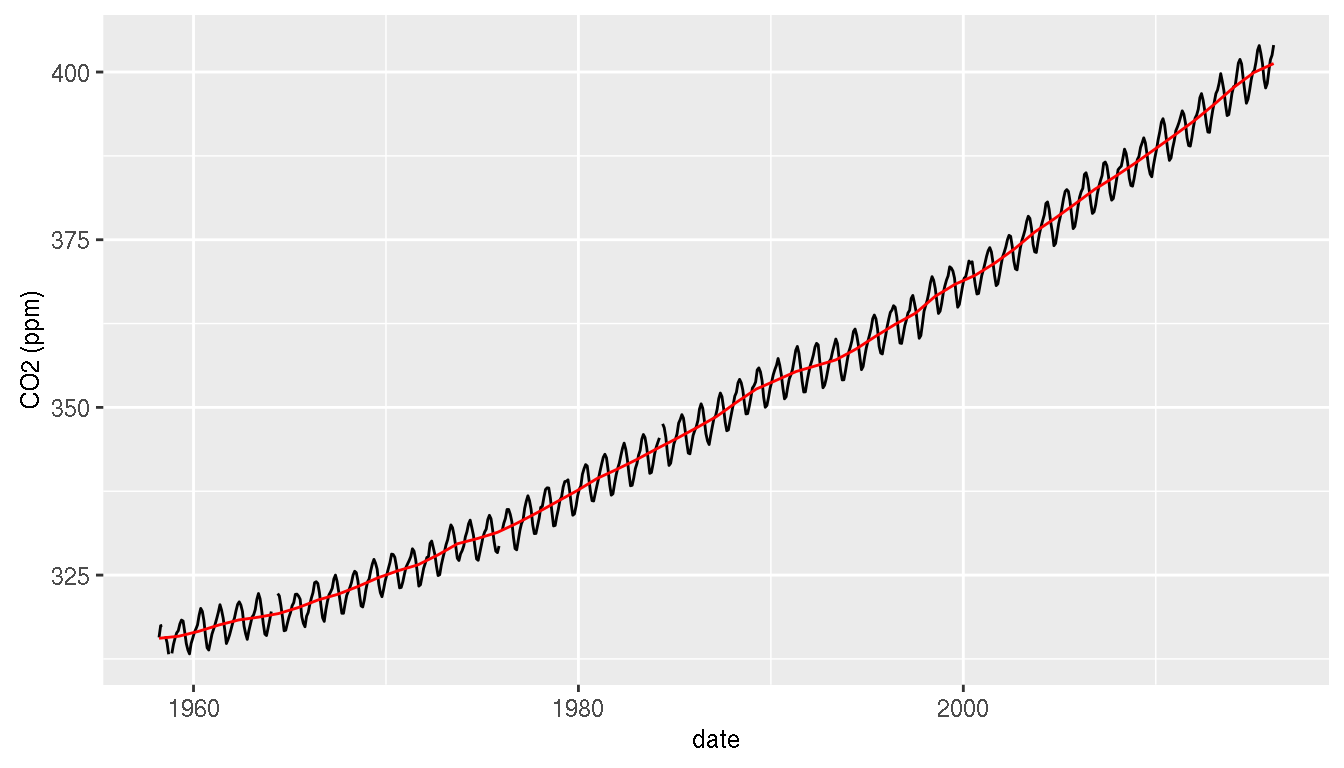# A visual introduction to rollply

``````library(proj4)
library(ggplot2)
library(rgdal)
library(tidyr)
library(rollply)
library(plyr) ``````

Rollply is a small function built upon plyr’s `ddply` function to facilitate moving-window-based computations. If you have a `data.frame`, give the dimensions over which the window should move, and rollply will make the subsets, apply the function on them and then combine the results into a data.frame.

In short, rollply extends the split-apply-combine strategy to moving-window computations, using a similar syntax. This tutorial thus assumes some basic familiarity with `plyr`, and in particular the function `ddply`. Let’s start with a simple example.

# Examples

## A time-series example

A simple use of moving-windows is adding a trendline to a time series plot. We will use the CO2 data from the Mauna Loa NOAA Observatory as a environmentally-conscious example.

``````# Download and format data
url <- "ftp://aftp.cmdl.noaa.gov/products/trends/co2/co2_mm_mlo.txt"
hawaii <- read.table(url)[ ,c(3,4)]
names(hawaii) <- c('date','CO2')
hawaii[hawaii\$CO2 < 0, "CO2"] <- NA # mark NAs as such

# Display original trend
CO2.plot <- ggplot(hawaii) + geom_line(aes(date, CO2)) + ylab("CO2 (ppm)")
print(CO2.plot)``````There is a clear trend here! Let’s smooth out the season effect (the wiggles in the black curve). We’ll use a window with a size of one year to compute a yearly average.

``````# with smoothed trend
hawaii.smoothed <- rollply(hawaii, ~ date, wdw.size = 1,
summarize, CO2.mean = mean(CO2, na.rm = TRUE), )
CO2.plot + geom_line(aes(date, CO2.mean), data = hawaii.smoothed, color = 'red')``````And voilà! A rather nice, although a bit depressing trend line for our data. When working on time-series, this represents an alternative to specialized packages such as `zoo` that also provide tools to apply functions over rolling windows.

Let’s take a more complex example that works on two-dimensional data.

## Exploring french town names

If you open a map of France, you’ll notice that towns and villages tend to have names that follow patterns. For example, Brittany’s towns are famous for having names starting with a “ker-”. Many towns in Lorraine end in “-ange” (a legacy from the german ending “-ingen”).

Can we visually explore the distribution of french towns names ? rollply can help here.

A moving-window approach essentially boils down to the following steps:

1. Build a grid over the whole country
2. For each point of the grid, take all the data poitns (towns) that are less than xx kilometers from it.
3. Check the names of the towns and count the ones matching a pattern. Return the number of matching towns.
4. Combine the results in a `data.frame`

Like `ddply` (in package `plyr`), `rollply` takes care of points 1,2 and 4. We just need to define a function that does number 3.

Let’s download a dataset of town names with their geographical coordinates:

``````# Download and prepare dataset
# Source and decription:
# https://publicdata.eu/dataset/listes-des-communes-par-rgions-dpartements-circonscriptions

tmpfile <- tempfile()
url <- paste0('http://www.nosdonnees.fr/wiki/images/b/b5/',
'EUCircos_Regions_departements_circonscriptions_communes_gps.csv.gz')
dat <- read.csv2(tmpfile, stringsAsFactors = FALSE)
file.remove(tmpfile)
dat <- dat[with(dat, latitude != "" | ! grepl(",", latitude)),
c('nom_commune', 'latitude', 'longitude')]
colnames(dat) <- c('name', 'latitude', 'longitude')

dat[ ,'name']      <- as.factor(tolower(dat[ ,'name']))
dat[ ,'latitude']  <- as.numeric(dat[ ,'latitude'])
dat[ ,'longitude'] <- as.numeric(dat[ ,'longitude'])

# We use an equirectangular projection to work on true distances
dat <- na.omit(dat)
dat <- data.frame(dat, proj4::project(dat[ ,c('longitude','latitude')],
'+proj=eqc'))
dat <- dat[ ,c('name','x','y')]``````
``````# Visualise distribution of towns
str(dat)``````
``````## 'data.frame':    33814 obs. of  3 variables:
##  \$ name: Factor w/ 34141 levels "aast","abainville",..: 1264 2487 2798 2821 3515 4005 4708 5689 5730 6645 ...
##  \$ x   : num  574507 585626 587480 561534 600452 ...
##  \$ y   : num  5146469 5159442 5152029 5155736 5129790 ...``````
``ggplot(dat) + geom_point(aes(x, y), alpha=.1)``